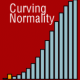# R-Sessions 31: Combining lmer output in a single table (UPDATED)

There are various ways of getting your output from R to your publication draft. Most of them are highly efficient, but unfortunately I couldn’t find a function that combines the output from several (lmer) models and presents it in a single table. lmer is the mixed effects model function from the lme4 package. So, I wrote a simple function that does exactly that.

Using it for a specific purpose, it is not a general function or something, but it can easily be adapted for use in other settings. Here it goes:

``` require(lme4) require(mlmRev) require(lme4) require(mlmRev)```

``` model.1 <- lmer(normexam ~ 1 + (1 | school), data=Exam) model.2 <- lmer(normexam ~ standLRT + (1 | school), data=Exam) model.3 <- lmer(normexam ~ standLRT + sex + (1 | school), data=Exam) model.4 <- lmer(normexam ~ standLRT + sex + schavg + (1 | school), data=Exam) model.a <- lmer(use ~ 1 + (1 | district), family=binomial, data=Contraception) model.b <- lmer(use ~ livch + (1 | district), family=binomial, data=Contraception) model.c <- lmer(use ~ age + (1 | district), family=binomial, data=Contraception) model.d <- lmer(use ~ livch + age + (1 | district), family=binomial, data=Contraception) m1 <- c(model.1, model.2, model.3, model.4) m2 <- c(model.a, model.b, model.c, model.d) combine.output.lmer <- function(models, labels=FALSE) { fix.coef <- lapply(models, function(x) summary(x)@coefs) var.coef <- lapply(models, function(x) summary(x)@REmat) n.par <- dim(summary(models[])@coefs) ifelse(labels==FALSE, fix.labels <- colnames(summary(models[])@coefs), fix.labels <- labels) var.labels <- colnames(var.coef[]) # Creating table with fixed parameters output.coefs <- data.frame(Row.names=row.names(fix.coef[])) for (i in 1:length(models)) { a <- fix.coef[[i]] colnames(a) <- paste("Model", i, fix.labels) output.coefs <- merge(output.coefs, a, by.x=1, by.y=0, all=T, sort=FALSE) } output.coefs[,1] <- as.character(output.coefs[,1]) output.coefs[dim(output.coefs)+2, 1] <- "Loglikelihood" LL <- unlist(lapply(models, function(x) as.numeric(logLik(x)))) output.coefs[dim(output.coefs), 1:length(models)*n.par-n.par+2] <- LL # Creating table with random parameters output.vars <- data.frame(var.coef[])[,1:2] for (i in 1:length(models)) { a <- var.coef[[i]] colnames(a) <- paste("Model", i, var.labels) output.vars <- merge(output.vars, a, by.x=1:2, by.y=1:2, all=T, sort=FALSE) } # Combining output.coefs and output.vars n.cols <- dim(output.coefs) n.coefs <- dim(output.coefs) n.vars <- dim(output.vars) output <- matrix(ncol=n.cols +1 , nrow=n.vars+n.coefs+2) output[1:n.coefs, -2] <- as.matrix(output.coefs) output[n.coefs+2, 1] <- "Variance Components" output[(n.coefs+3) : (n.coefs+n.vars+2), 1:2] <- as.matrix(output.vars[,1:2]) output[ (n.coefs+3) : (n.coefs+n.vars+2), which(rep(c(1,1,rep(0, n.par-2)),length(models))!=0)+2] <- as.matrix(output.vars[,c(-1,-2)]) colnames(output) <- c("Parameter", "Random", colnames(output.coefs)[-1]) return(output) } combined <- combine.output.lmer(m1) combined <- combine.output.lmer(m2) combined <- combine.output.lmer(m1, labels=c("appel", "banaan", "grapefruit")) combined <- combine.output.lmer(m2, labels=c("appel", "peer", "banaan", "grapefruit")) ```

```write.csv(combined, "combined.csv", na=" ") ```

In this example I estimate four mixed effects models, which are concatenated in a single object 'm'. The function itself is called 'combine.output.lmer', and is used on the object 'm'. The output is a data.frame with the variable names in the first column. Not-estimated parameters in models are indicated by 'NA' in their respective columns. By writing the 'combined'-object to an external file, the NA's are lost and the file can be read into other software, such as Open Office Spreadsheet or Excell. Use the xtable-package to get it in your latex document.

UPDATE
I updated and improved the code somewhat, for I wasn't satisfied with the results. Now the code adapts to the number of parameters derived form the models' summary, allows to add your own names to the columns, and, most importantly, also reports the random slopes.

Please note: due to the internal matching procedure, errors may occur when the same variable is random 'within' more than one other variable. This is only the case when other variables are random within each nesting factor as well.

## 4 comment on “R-Sessions 31: Combining lmer output in a single table (UPDATED)”

•I get an error in your code here:

> ifelse(labels==FALSE,
+ fix.labels <- colnames(summary(models[])@coefs),
+ fix.labels |z|)”

•Vivyan Cyril

Can you post how the table looks like?

•Hi!

I could, of course, provide an example of the output, but in fact I would recommend to use the stargazer package: https://cran.r-project.org/web/packages/stargazer/index.html . This is an old post, and the function was just a quick fix for a problem I was dealing with back then. Now, much better solutions are available, including the aforementioned stargazer.